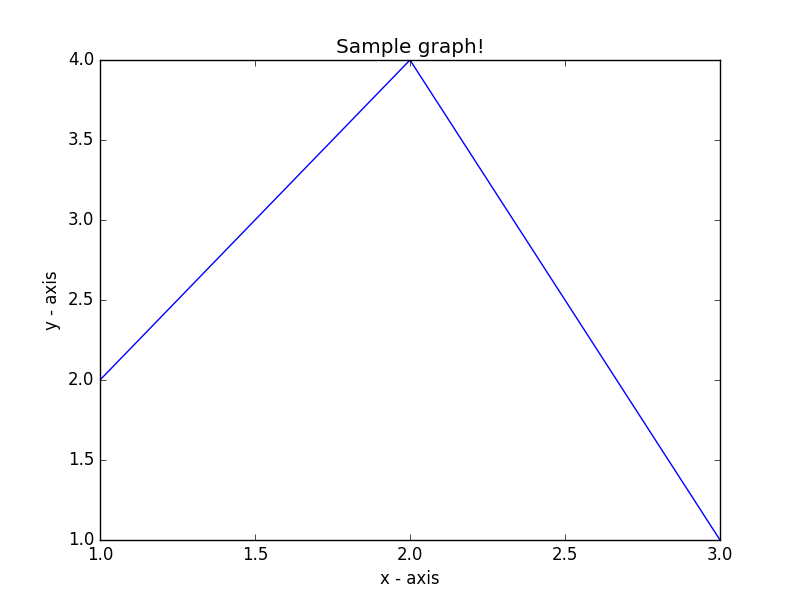﻿ Matplotlib Basic: Draw a line using given axis values taken from a text file, with suitable label in the x axis, y axis and a title - w3resource# Matplotlib Basic: Draw a line using given axis values taken from a text file, with suitable label in the x axis, y axis and a title

## Matplotlib Basic: Exercise-3 with Solution

Write a Python program to draw a line using given axis values with suitable label in the x axis , y axis and a title.
test.txt
1 2
2 4
3 1

Sample Solution:

Python Code:

``````import matplotlib.pyplot as plt
with open("test.txt") as f:
data = data.split('\n')
x = [row.split(' ') for row in data]
y = [row.split(' ') for row in data]
plt.plot(x, y)
# Set the x axis label of the current axis.
plt.xlabel('x - axis')
# Set the y axis label of the current axis.
plt.ylabel('y - axis')
# Set a title
plt.title('Sample graph!')
# Display a figure.
plt.show()
```
```

Sample Output:Python Code Editor: